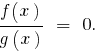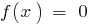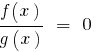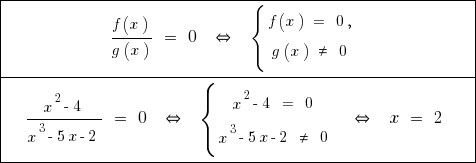# Fractional equations, how razvesti fractional equation

## Scheme the solution of fractional equations

1. The use of equations and effect

1. Bring to mind2. Find the roots of the equation3. Perform background checks on substitution in the original equation
2. Using the properties of the relevant functions
3. The use of equivalent transformations
1. Fix odz initial equation
2. Bring to mindand Rozvadov these equations by the original DHS or performed the equivalent transformation to introduce a convenient change of variables.
3. Perform background checks on substitution in the original equation

## Equivalent transformation of simple fractional equationsTags:
Chapter:
Versions in other languages:
Share with friends: